## How to Calculate and Solve for Maximum Velocity to avoid Overturning of a Vehicle moving along a Level Circular Path | The Calculator Encyclopedia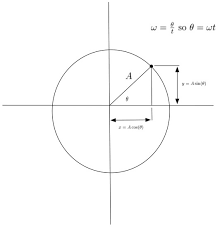The image above represents the maximum velocity to avoid overturning of a vehicle moving along a level circular path.

To compute for the maximum velocity, four essential parameters are needed and these parameters are Acceleration due to Gravity (g), Height of Centre of Gravity of the Vehicle from Ground Level (h), Radius of Circular Path (r) and Half of the Distance between the Centre Lines of the Wheel (a).

The formula for calculating the maximum velocity:

vmax = √(gra / h)

Where:
vmax = Maximum Velocity to avoid Overturning of a Vehicle moving along a Level Circular Path
g = Acceleration due to Gravity
h = Height of Centre of Gravity of the Vehicle from Ground Level
r = Radius of Circular Path
a = Half of the Distance between the Centre Lines of the Wheel

Let’s solve an example;
Find the maximum velocity when the Acceleration due to Gravity (g) is 10.2, Height of Centre of Gravity of the Vehicle from Ground Level (h) is 14, Radius of Circular Path (r) is 22 and Half of the Distance between the Centre Lines of the Wheel (a) is 32.

This implies that;
g = Acceleration due to Gravity = 10.2
h = Height of Centre of Gravity of the Vehicle from Ground Level = 14
r = Radius of Circular Path = 22
a = Half of the Distance between the Centre Lines of the Wheel = 32

vmax = √(gra / h)
vmax = √((10.2)(22)(32)/14)
vmax = √((7180.79)/14)
vmax = √(512.91)
vmax = 22.647

Therefore, the maximum velocity to avoid Overturning of a Vehicle moving along a Level Circular Path is 22.647 m/s.

## How to Calculate and Solve for the Reaction at the Inner Wheel of a Vehicle moving along a Level Circular Path | The Calculator Encyclopedia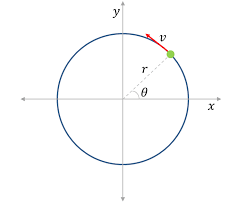The image represents reaction at the inner wheel of a vehicle moving along a level circular path.

To compute for the reaction, six essential parameters are needed and these parameters are Mass of the Vechicle (m), Acceleration due to Gravity (g), Velocity of the Vehicle (v), Height of Centre of Gravity of the Vehicle from Ground Level (h), Radius of Circular Path (r) and Half of the Distance between the Centre Lines of the Wheel (a).

The formula for calculating the reaction at the inner wheel of a vehicle moving along a level circular path:

RA = mg / 2[1 – v²h / gra]

Where:
RA = Reaction at the Inner Wheel of a Vehicle moving along a Level Circular Path
m = Mass of the Vechicle
g = Acceleration due to Gravity
v = Velocity of the Vehicle
h = Height of Centre of Gravity of the Vehicle from Ground Level
r = Radius of Circular Path
a = Half of the Distance between the Centre Lines of the Wheel

Let’s solve an example;
Find the reaction when Mass of the Vechicle (m) is 13, Acceleration due to Gravity (g) is 9.8, Velocity of the Vehicle (v) is 11, Height of Centre of Gravity of the Vehicle from Ground Level (h) is 5, Radius of Circular Path (r) is 7 and Half of the Distance between the Centre Lines of the Wheel (a) is 3.

This implies that;
m = Mass of the Vechicle = 13
g = Acceleration due to Gravity = 9.8
v = Velocity of the Vehicle = 11
h = Height of Centre of Gravity of the Vehicle from Ground Level = 5
r = Radius of Circular Path = 7
a = Half of the Distance between the Centre Lines of the Wheel = 3

RA = mg / 2[1 – v²h / gra]
RA = 13(9.8) / 2[1 – (11)²(5) / (9.8)(7)(3)]
RA = 127.4 / 2[1 – (121)(5) / 205.8]
RA = 63.7[1 – 605 / 205.8]
RA = 63.7[1 – 2.939]
RA = 63.7[-1.939]
RA = -123.56

Therefore, the reaction at the inner wheel of a vehicle moving along a level of circular path is -123.56 N.

## How to Calculate and Solve for the Reaction at the Outer Wheel of a Vehicle moving along a Level Circular Path | Nickzom Calculator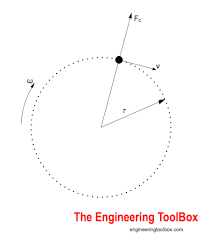The image represents reaction at the outer wheel of a vehicle moving along a level circular path.

To compute for the reaction, six essential parameters are needed and these parameters are Mass of the Vechicle (m), Acceleration due to Gravity (g), Velocity of the Vehicle (v), Height of Centre of Gravity of the Vehicle from Ground Level (h), Radius of Circular Path (r) and Half of the Distance between the Centre Lines of the Wheel (a).

The formula for calculating the reaction at the outer wheel of a vehicle moving along a level circular path:

RB = mg / 2[1 + v²h/gra]

Where;
RB = Reaction at the Outer Wheel of a Vehicle moving along a Level Circular Path
m = Mass of the Vechicle
g = Acceleration due to Gravity
v = Velocity of the Vehicle
h = Height of Centre of Gravity of the Vehicle from Ground Level
r = Radius of Circular Path
a = Half of the Distance between the Centre Lines of the Wheel

Let’s solve an example;
Find the reaction when Mass of the Vechicle (m) is 12, Acceleration due to Gravity (g) is 9.8, Velocity of the Vehicle (v) is 28, Height of Centre of Gravity of the Vehicle from Ground Level (h) is 16, Radius of Circular Path (r) is 8 and Half of the Distance between the Centre Lines of the Wheel (a) is 4.

This implies that;
m = Mass of the Vechicle = 12
g = Acceleration due to Gravity = 9.8
v = Velocity of the Vehicle = 28
h = Height of Centre of Gravity of the Vehicle from Ground Level = 16
r = Radius of Circular Path = 8
a = Half of the Distance between the Centre Lines of the Wheel = 4

RB = mg / 2[1 + v²h/gra]
RB = 12 x 9.8 / 2[1 + 28² x 16/9.8 x 8 x 4]
RB = 117.60 / 2[1 + 784 x 16/313.6]
RB = 58.80[1 + 40]
RB = 58.80
RB = 58.80
RB = 2410.8

Therefore, the reaction of the outer wheel of a vehicle moving along a level circular path is 2410.8 N.

## How to Calculate and Solve for Mass, Height and Potential Energy | The Calculator Encyclopedia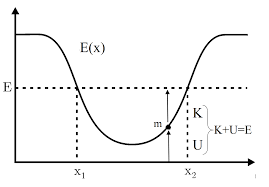The image above represents potential energy.

To compute for the potential energy, three essential parameters are needed and these parameters are mass (m), height (h) and acceleration due to gravity (g).

The formula for calculating the potential energy:

P.E = mgh

Where;
P.E. = Potential Energy
m = Mass
g = acceleration due to gravity
h = Height

Let’s solve an example;
Find the potential energy when the mass is 12 with a height of 24 and acceleration due to gravity of 9.8.

This implies that;
m = Mass = 12
g = acceleration due to gravity = 9.8
h = Height = 24

P.E = mgh
P.E = 12 x 9.8 x 24
P.E = 2822.4

Therefore, the potential energy is 2822.4 Joules (J).

Calculating the Mass when Potential Energy, Height and Acceleration due to Gravity is Given.

m = P.E / gh

Where;
m = Mass
P.E. = Potential Energy
g = acceleration due to gravity
h = Height

Let’s solve an example;
Find the Mass when potential energy is 450 with a height of 30 and acceleration due to gravity of 10.

This implies that;
P.E. = Potential Energy = 450
g = acceleration due to gravity = 10
h = Height = 30

m = P.E / gh
m = 450 / 10 x 30
m = 450 / 300
m = 1.5

Therefore, the mass is 1.5.

## How to Calculate and Solve for Escape Velocity | The Calculator Encyclopedia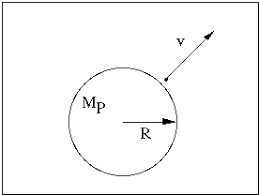The image above represents the escape velocity.

To compute the escape velocity of a field, two essential parameters are needed and the parameters are acceleration due to gravity (g) and radius (r).

The formula for calculating the escape velocity:

V = √(2gR)

Where;
V = Escape velocity
g = Acceleration due to gravity
R = Radius

Let’s solve an example;
Find the escape velocity of a field when the acceleration due to gravity is 12 and the radius is 24 cm.

This implies that;
g = Acceleration due to gravity = 12
r = Radius = 24 cm

V = √(2gR)
V = √(2 x 12 x 24)
V = √(576)
V = 24

Therefore, the escape velocity is 24 m/s.

## Nickzom Calculator Calculates Range and Maximum Range of a Projectile in Physics

To get the answer for the range of a projectile, there are three essential parameters to be given which are:

• Acceleration due to Gravity
• Initial Velocity
• Angle of Projection

Where as to get the answer for maximum range of a projectile requires two parameters to be given which are:

• Acceleration due to Gravity
• Initial Velocity

Let’s first, proceed to the home page of the app. Select Physics and click on Open SectionThen proceed to click on Projectile## Nickzom Calculator Calculates Maximum Height of a Projectile

According to SoftSchools.com,

A projectile is an object that is given an initial velocity, and is acted on by gravity. The maximum height of the object is the highest vertical position along its trajectory. The maximum height of the projectile depends on the initial velocity v0, the launch angle θ, and the acceleration due to gravity. The unit of maximum height is meters (m).

Nickzom Calculator calculates the maximum height of a projectile given the accurate values for the required parameters. The required parameters to get the answer to maximum height are:

• Acceleration due to Gravity
• Initial Velocity
• Angle of Projection

Let’s get started, first and foremost, proceed to the home page of the app, select Physics and click on Open Section.## Nickzom Calculator Calculates the Total Time of Flight and Time of Flight at Maximum Height in Projectile (Physics)

I am not hear to start explaining to you the meaning and application of time of flight. I believe you are here to know how the calculator encyclopedia calculates these parameters.

Nickzom Calculator requires three parameters to get the answer for time of flight and these parameters are:

• Acceleration due to Gravity
• Initial Velocity
• Angle of Projection

Let’s first get the workings and answer for time of flight at maximum height where the acceleration due to gravity is 10 m/s2, initial velocity is 20 m/s and angle of projection is 30°.

First and Foremost, Select Physics from the home page and click on Open SectionIn the Physics section, Click on Projectile